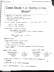# STAT 1100Q Lecture 11: Inference 1

46 views6 pagesturquoisehedgehog153 and 1 other unlocked2
Verified Note
2 documents

## Document Summary

Statistics a number a number that that describes a population is computed from the sample. 0. 46 n a 500 population adults aged 18 29 p o 14. 5 purpose of inference parameters are usually unknown. Cpopulation is too large so we calculate statistics from properly conducted samples to estimate the parameters. 6 due to sampling variability it implies that no two random samples are the same so since statistics are calculated from samples statistics also vary from sample to sample. 7 unusual more than two standard deviations above or below the mean. 8 sampling distribution of sample proportions population n225. Shape e sz i i i na 25 pz 12. If np 3 10 d n l p 310. Nci p a 928 i 0. 56 310 so approximately normal center mn p p mean of sample proportions population proportion. C z score x e p mp op. 0. 016 reasonable d p p 7 0. 52 z p z 7 0. 52 0. 56.

## Get access

\$8 USD/m\$10 USD/m
Billed \$96 USD annuallyHomework Help
Study Guides
Textbook Solutions
Class Notes
Textbook Notes
Booster Class
Class+
\$8 USD/m
Billed \$96 USD annuallyHomework Help
Study Guides
Textbook Solutions
Class Notes
Textbook Notes
Booster Class